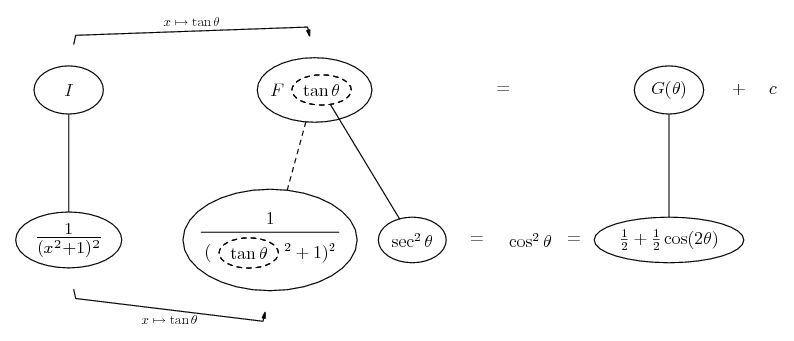# Thread: show the way

1. ## show the way

How does one carry out

$\displaystyle \int\frac{1}{(x^2+1)^2}dx$

2. - Wolfram|Alpha

Click on 'show steps'

Edit:

Just in case a picture helps...... where... is the chain rule. Straight continuous lines differentiate downwards (integrate up) with respect to x, and the straight dashed line similarly but with respect to the dashed balloon expression (the inner function of the composite which subject to the chain rule).

Carry on the anti-clockwise journey (to I) by finding G(theta) and mapping back from tan(theta) to x.

__________________________________________

Don't integrate - balloontegrate!

http://www.ballooncalculus.org/examples/gallery.html

http://www.ballooncalculus.org/asy/doc.html

3.Originally Posted by nthethemHow does one carry out

$\displaystyle \int\frac{1}{(x^2+1)^2}dx$
What Tom@ballooncalculus is saying, in his own unique way, is that, since $\displaystyle tan^2(u)+ 1= set^2(u)$, use the substitution tan(u)= x.